# allrite

## Strange Attractors – IFS FractalsAlong with the Mandelbrot set and Lorentz’s butterfly, the fractal fern is one of the best known fractal. It was created by Michael F. Barnsley and is an example of an Iterated Function System (IFS) fractal. IFS fractals are created by using affine transformations. A two-dimensional affine transformation on the Euclidean plane is given by BarnsleyThis consists of a linear transformation performed by the matrix containing a to d and then a translation by the matrix [e,f].

### Fractals as Contraction Maps

In general, following on from Hutchinson, the term similitudes will be used instead of affine transformation, although the latter is more common. A similitude is any map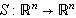(where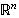is the space of the n-dimensional reals) which can be expressed in the form of a composition of a dilation, an orthonormal transformation and a translation Hutchinson. A dilation with a fixed point and dilation ratio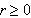is a map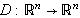of the form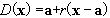. An orthonormal transformation is a linear transformation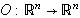such that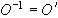. For example, in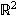and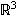the maps would be a rotation and followed possibly by a reflection. A translation is a map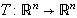of the form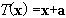.

Hutchinson defines a fractal into be a compact set K equal to a finite union of similitudes,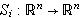acting on K, for a family of similitudes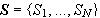. In fact, the similitudes can be replaced by a family of contraction maps on.

A contraction map is one which maps two points closer together. That is, if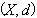is a metric space and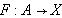, where A is a subset of X, F is a contraction map, and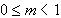then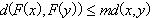for all x and y in X.

Now, ifis a family of contraction maps onthen it can be proven (See Hutchinson) that there is a unique compact set K such that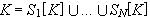.

Applying further analysis to the problem it turns out that S itself is a contraction map and by the Contraction Mapping Theorem that K is actually the fixed point of S. So if we iteratively apply the contractive map S to a compact subset A of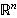then the points of A will be attracted to the fractal set K. In other words, like the attractors of the chaotic non-linear pendulum, K is a strange attractor of the map S, and what is more is that it is the only attractor. This is the basis of an IFS fractal.

The Koch snowflake is an example of this. It is generated by a union of four similitudes. All begin with a dilation of 1/3. S2 composes this with a translation, then a rotation by 60 degrees in the anti-clockwise direction. S3 is instead rotated in the clockwise direction, while S4 is only a dilation and translation. The same four affine transformations are continued in the next iteration and so on, making the curve ever more complex.### Random Fractals

In practice, IFS fractals are almost always drawn by using a probabilistic technique. Each of the similitudes is assigned a probability. With each iteration, the transformation to be used is then a random selection, with the chances that a particular one will be used depending on the probabilities assigned. This sequence is still attracted by the same attractor and will hence converge to the same fractal as would be obtained by using the non-random method.

An illustration of the above theory can be obtained using Barnsley’s Desktop Fractal Design System software. The first image is of a Sierpinski gasket. The three smaller boxes in the image correspond with the three affine transformations used to create the fractal. Using the notation first introduced above for affine transformations we can tabulate the values for the three attractors:

a

b

c

d

e

f

Probability

50

0

0

50

0

0

1/3

50

0

0

50

128

0

1/3

50

0

0

50

64

80

1/3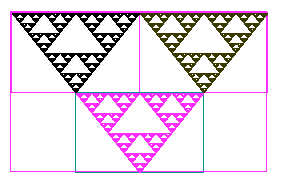Suppose we modify the attractors now and see what happens to the image. The first change we make is to change the translation factor of one transformation. That is, we shift it by a bit. As can be seen, the resulting attractor is still easily recognisable as a Sierpinski gasket. Nevertheless, it is different to the original as it is skewed.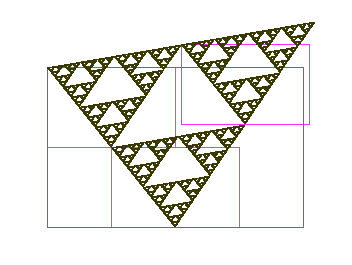Starting again with the original factors of the Sierpinski fractal, the second modification was to change the dilation ratio of the bottom attractor. The resulting fractal now takes on a very different appearance with the triangular holes now looking like Christmas trees.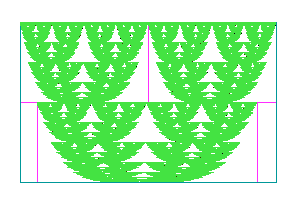The most remarkable change occurs when the leftmost attractor was rotated through about 100 degrees in the clockwise direction.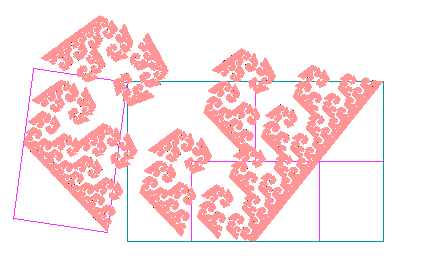I’m not sure how to describe this one!

These examples serve to demonstrate the unique relationship between a fractal and its generating similitudes. It is also possible to find the appropriate affine transformations for a given fractal. This is the principle behind fractal encoding of photographs. For more information on this visit Barnsley’s Iterated Systems Inc website.